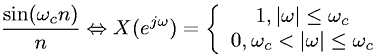Equations > Signal Processing > Fourier Transform Pairs > Discrete-Time Fourier transform of a sinc sequence

### Discrete-Time Fourier transform of a sinc sequenceLatex Code:

MathML Code:

 $\frac\mathrm{sin}\left({\omega }_{c}n\right)n⇔X\left({e}^{j\omega }\right)=\left\{\begin{array}{c}\hfill 1,|\omega |\le {\omega }_{c}\hfill \\ \hfill 0,{\omega }_{c}<|\omega |\le {\omega }_{c}\hfill \end{array}$

MathType 5.0: